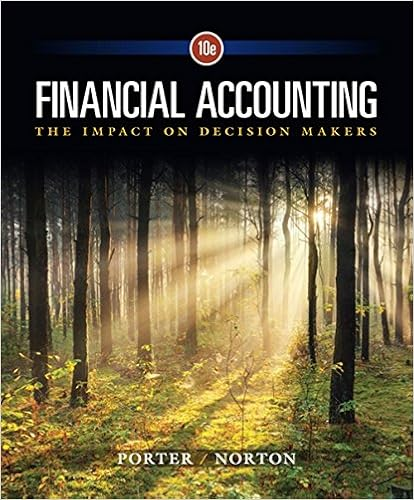# New common stock is more expensive than k e a to

• Test Prep
• ProfLightningSkunk5289
• 60
• 93% (68) 63 out of 68 people found this document helpful

This preview shows page 13 - 16 out of 60 pages.

##### We have textbook solutions for you!
The document you are viewing contains questions related to this textbook.The document you are viewing contains questions related to this textbook.
Chapter 13 / Exercise 1
Financial Accounting: The Impact on Decision Makers
Norton/PorterExpert Verified
73. New common stock is more expensive than KeA. to compensate for risk.B. to compensate for more dividends.C. to compensate for expansionary problems.D. to cover distribution costs.
74. In computing the cost of common equity, if D1 goes downward and Pogoes up, Kewill
75. In determining the cost of retained earnings
11-13
##### We have textbook solutions for you!
The document you are viewing contains questions related to this textbook.The document you are viewing contains questions related to this textbook.
Chapter 13 / Exercise 1
Financial Accounting: The Impact on Decision Makers
Norton/PorterExpert Verified
Chapter 11 - Cost of Capital76. Within the capital asset pricing model
77. Using the constant growth model, a firm's expected (D1) dividend yield is 4% of the stock price, and its growth rate is 5%. If the tax rate is .35%, what is the firm's cost of equity? A. 10%B. 6.65%C. 9.0%D. More information is required
78. Expected cash dividends are \$3.00, the dividend yield is 4%, flotation costs are 4% of price, and the growth rate is 3%. Compute cost of new common stock.
79. A firm's stock is selling for \$65. The dividend yield is 6%. A 7% growth rate is expected for the common stock. The firm's tax rate is 40%. What is the firm's cost of retained earnings?
80. A firm's stock is selling for \$62. The next annual dividend is expected to be \$3.00. The growth rate is 9%. The flotation cost is \$5.00. What is the cost of retained earnings?
11-14
Chapter 11 - Cost of Capital81. For many firms, the cheapest and most important source of equity capital is in the form of A. debt.B. common stock.C. preferred stock.D. retained earnings.
82. Retained earnings has a cost associated with it because:
83. There may be a change in the marginal cost of capital curve because
84. The after-tax cost of debt will almost always be below
•••